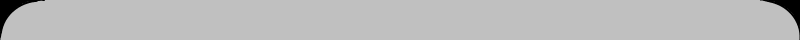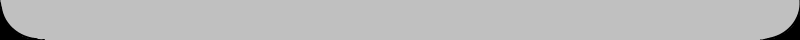8. Working with random numbers

When you make a game, you wouldn't normally want it to go the same way every time it is played. A good way to get some variation into a game is by making use of so-called random numbers.

In Java you can make a "random" number with the help of the method random(), which is part of the Math class. This method returns a random (more or less unpredictable) broken number which is at least 0 and smaller than 1. We could produce such a random number r in the following way:

double r = Math.random();

After this line, r could (for instance) have thefollowing value:

0.4303072576758019

By the way, double is a type specially used for broken numbers which must be very accurate. A variable of the double type has a very large precision.

But let's return to our random number r. Sometimes you don't want broken numbers, but something like a number produced by throwing dice (typically, the numbers 1 through 6). Let's try to emulate the throw of a die. We can multiply the expression above by 6:

double r = 6 * Math.random(); // 0 - 5.999...

The number produced is still a broken number (or floating point number, as it is called) and its minimum is still 0, but its maximum is almost 6 now (5 point .....).

It is possible to cut off the fraction part of this number with a so-called cast. A cast looks like a type in parentheses, for instance like this: (char)a or (int)b.

As we saw before, it is used to change one type of variable into another one. In this case we can use this cast:

int r = (int)(6*Math.random()); // 0,1,2,3,4,5

What we are left with is a number which is at least 0 and whose maximum is 5. The numbers on a die, however, are at least 1 and have a maximum of 6. We can obtain this by simply adding 1, so:

int die = (int)(6*Math.random()) + 1;  // 1,2,3,4,5,6

Now that we can make random numbers, we could put together a simple game. The game we are going to make works like this: the program takes "in mind" a random number (1 through 100), and the player may try to to find out what number the program "thinks of". After each guess, the program will issue one of only three answers too high, too low and correct.

The program has a number of well-defined stages:

2. guessing the number, while it is too high or too low
3. number is correct; game can start again

In order to know which stage the program is in, we shall use a variable called round, which will be increased every round. We could check the value of round like this:

if (round == 1)
text = "This is round 1";
else if (round == 2)
text = "This is round 2";
else
text = "Other round";

But for cases like this, Java has a construction which is shorter, easier to use and which looks neater as well. This is what it looks like:

switch (round) {
case 1: text = "This is round 1"; break;
case 2: text = "This is round 2"; break;
default: text = "Other round";
}

When the program encounters a 'hit', all the following lines would be executed. This is prevented by the addition of break. The expression default refers to all other values, in this case all other values than 1 and 2.

We can use another variable times to count the number of guesses needed, so that after the game we can issue a statement like:

Number found in 7 times.

When the player has to type in a new number, it would be handy if the cursor is already in the textfield and the number typed previously does not have to be erased first. This can be done with:

textField.setText(""); // put an empty string in the textfield
textField.requestFocus();// puts the cursor in the textfield

If the player wants to play again, it would be nice if the new number is not thesame as the number that was used in the previous game. This can be done like this:

do {
secret = 1 + (int)(100 * Math.random());
} while (secret == x);

And here is the complete program:

import java.applet.*;
import java.awt.*;
public class Guessing extends Applet {
TextField field;
Button button;
String text="I'll make up a number (1-100)";
int round = 1, times, x, secret;
public void init() {
setBackground (Color.white);
field = new TextField(10);
button = new Button ("Click");
secret = 1+(int)(100*Math.random());
}
public void paint (Graphics g) {
g.drawString (text,40,100);
g.drawString ("Click to continue",40,160);
field.setText("");
field.requestFocus();
}
public boolean action (Event e, Object o) {
if (e.target == button) {
round++;
switch(round) {
case 1:
text = "I'll make up a number (1-100)";break;
case 2:
text = "And will you try to find it."; break;
case 3:
text = "Type in a number ..."; break;
default:
try {
String word = field.getText();
x = Integer.parseInt(word);
} catch(java.lang.NumberFormatException exc){
text = "no correct number ... ";
repaint();
return false;
}
times++;
if (x > secret)
text = x+" is too high, please try again.";
else if (x < secret)
text = x+" is too low, please try again.";
else {
text = x+" found in " + times + " times.";
round = times = 0;
do {
secret = 1+(int)(100*Math.random());
} while (x == secret);
}
}
repaint();
return true;
}
return false;
}
}

Exercise 8.1

Please write an applet in which you see a new proverb after each click (at least five times) on a button. After the last proverb the program starts again with proverb no 1.

Exercise 8.2

Please rewrite the Guessing applet so that the roles are reversed: the player takes a number in mind and the computer must try to find it. You could use three buttons called Higher, Lower and Correct. If the player tries to cheat, the game must be ended (see the example below).

• To chapter 9
•(c) 2003-2008, Thomas J.H.Luif